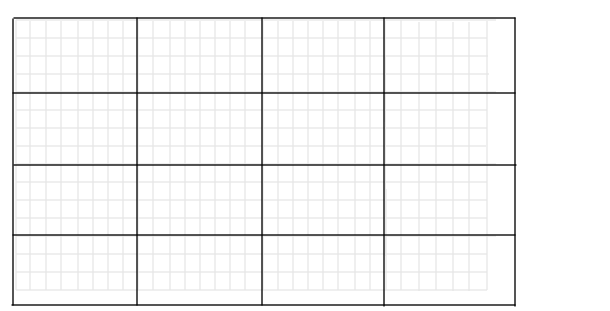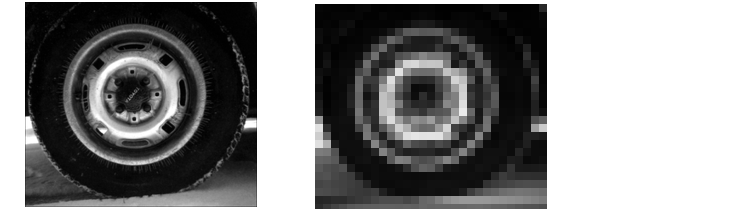Image Processing Toolbox User's GuideDistinct Block Operations

Distinct blocks are rectangular partitions that divide a matrix into m-by-n sections. Distinct blocks overlay the image matrix starting in the upper left corner, with no overlap. If the blocks don't fit exactly over the image, the toolbox adds zero padding so that they do. The following figure shows a 15-by-30 matrix divided into 4-by-8 blocks.Image Divided into Distinct Blocks

The zero padding process adds 0's to the bottom and right of the image matrix, as needed. After zero padding, the matrix is size 16-by-32.

The function `blkproc` performs distinct block operations. blkproc extracts each distinct block from an image and passes it to a function you specify. blkproc assembles the returned blocks to create an output image.

For example, the command below processes the matrix `I` in 4-by-6 blocks with the function `myfun`. (For more information about using function handles, see `function_handle` in the MATLAB Function Reference documentation.)

• ```I2 = blkproc(I,[4 6],@myfun);
```

You can specify the function as an inline function. For example:

• ```f =  inline('mean2(x)*ones(size(x))');
I2 = blkproc(I,[4 6],f);
```

The example below uses `blkproc` to set every pixel in each 8-by-8 block of an image matrix to the average of the elements in that block.

• ```I = imread('tire.tif');
f = inline('uint8(round(mean2(x)*ones(size(x))))');
I2 = blkproc(I,[8 8],f);
imshow(I)
figure, imshow(I2);```

Notice that `inline` computes the mean of the block and then multiplies the result by a matrix of ones, so that the output block is the same size as the input block. As a result, the output image is the same size as the input image. `blkproc` does not require that the images be the same size; however, if this is the result you want, you must make sure that the function you specify returns blocks of the appropriate size.Linear and Nonlinear Filtering Overlap